# Text in Matplotlib Plots¶

Introduction to plotting and working with text in Matplotlib.

Matplotlib has extensive text support, including support for mathematical expressions, truetype support for raster and vector outputs, newline separated text with arbitrary rotations, and unicode support.

Because it embeds fonts directly in output documents, e.g., for postscript or PDF, what you see on the screen is what you get in the hardcopy. FreeType support produces very nice, antialiased fonts, that look good even at small raster sizes. Matplotlib includes its own matplotlib.font_manager (thanks to Paul Barrett), which implements a cross platform, W3C compliant font finding algorithm.

The user has a great deal of control over text properties (font size, font weight, text location and color, etc.) with sensible defaults set in the rc file. And significantly, for those interested in mathematical or scientific figures, Matplotlib implements a large number of TeX math symbols and commands, supporting mathematical expressions anywhere in your figure.

## Basic text commands¶

The following commands are used to create text in the pyplot interface and the object-oriented API:

pyplot API OO API description
text text Add text at an arbitrary location of the Axes.
annotate annotate Add an annotation, with an optional arrow, at an arbitrary location of the Axes.
xlabel set_xlabel Add a label to the Axes's x-axis.
ylabel set_ylabel Add a label to the Axes's y-axis.
title set_title Add a title to the Axes.
figtext text Add text at an arbitrary location of the Figure.
suptitle suptitle Add a title to the Figure.

All of these functions create and return a Text instance, which can be configured with a variety of font and other properties. The example below shows all of these commands in action, and more detail is provided in the sections that follow.

import matplotlib
import matplotlib.pyplot as plt

fig = plt.figure()
ax = fig.add_subplot(111)
fig.subplots_adjust(top=0.85)

# Set titles for the figure and the subplot respectively
fig.suptitle('bold figure suptitle', fontsize=14, fontweight='bold')
ax.set_title('axes title')

ax.set_xlabel('xlabel')
ax.set_ylabel('ylabel')

# Set both x- and y-axis limits to [0, 10] instead of default [0, 1]
ax.axis([0, 10, 0, 10])

ax.text(3, 8, 'boxed italics text in data coords', style='italic',
bbox={'facecolor': 'red', 'alpha': 0.5, 'pad': 10})

ax.text(2, 6, r'an equation: $E=mc^2$', fontsize=15)

ax.text(3, 2, 'unicode: Institut für Festkörperphysik')

ax.text(0.95, 0.01, 'colored text in axes coords',
verticalalignment='bottom', horizontalalignment='right',
transform=ax.transAxes,
color='green', fontsize=15)

ax.plot(, , 'o')
ax.annotate('annotate', xy=(2, 1), xytext=(3, 4),
arrowprops=dict(facecolor='black', shrink=0.05))

plt.show()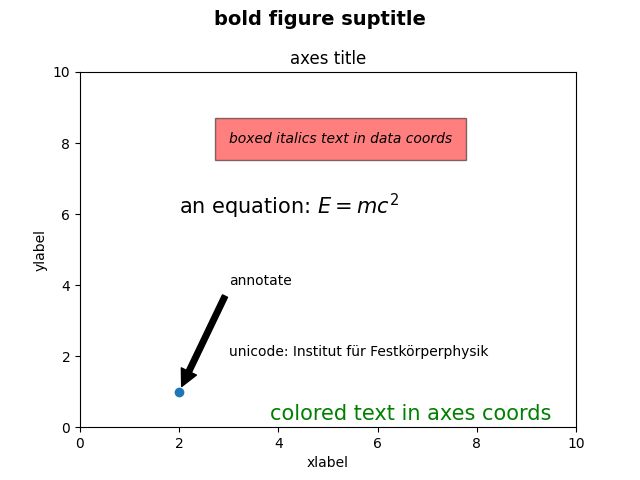## Labels for x- and y-axis¶

Specifying the labels for the x- and y-axis is straightforward, via the set_xlabel and set_ylabel methods.

import matplotlib.pyplot as plt
import numpy as np

x1 = np.linspace(0.0, 5.0, 100)
y1 = np.cos(2 * np.pi * x1) * np.exp(-x1)

fig, ax = plt.subplots(figsize=(5, 3))
fig.subplots_adjust(bottom=0.15, left=0.2)
ax.plot(x1, y1)
ax.set_xlabel('time [s]')
ax.set_ylabel('Damped oscillation [V]')

plt.show()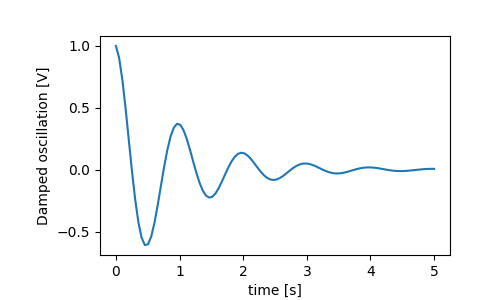The x- and y-labels are automatically placed so that they clear the x- and y-ticklabels. Compare the plot below with that above, and note the y-label is to the left of the one above.

fig, ax = plt.subplots(figsize=(5, 3))
fig.subplots_adjust(bottom=0.15, left=0.2)
ax.plot(x1, y1*10000)
ax.set_xlabel('time [s]')
ax.set_ylabel('Damped oscillation [V]')

plt.show()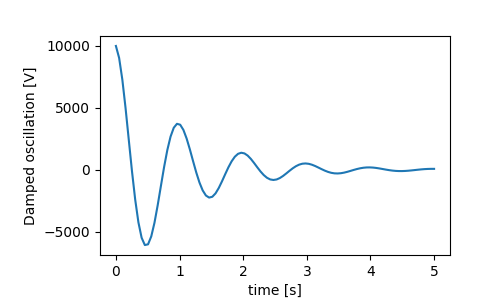If you want to move the labels, you can specify the labelpad keyword argument, where the value is points (1/72", the same unit used to specify fontsizes).

fig, ax = plt.subplots(figsize=(5, 3))
fig.subplots_adjust(bottom=0.15, left=0.2)
ax.plot(x1, y1*10000)
ax.set_xlabel('time [s]')
ax.set_ylabel('Damped oscillation [V]', labelpad=18)

plt.show()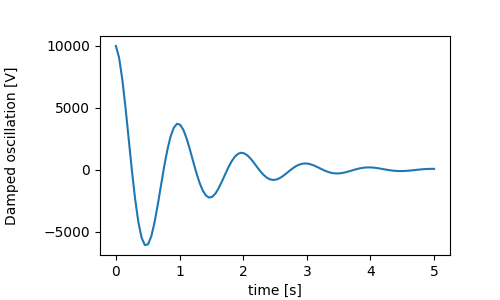Or, the labels accept all the Text keyword arguments, including position, via which we can manually specify the label positions. Here we put the xlabel to the far left of the axis. Note, that the y-coordinate of this position has no effect - to adjust the y-position we need to use the labelpad kwarg.

fig, ax = plt.subplots(figsize=(5, 3))
fig.subplots_adjust(bottom=0.15, left=0.2)
ax.plot(x1, y1)
ax.set_xlabel('time [s]', position=(0., 1e6), horizontalalignment='left')
ax.set_ylabel('Damped oscillation [V]')

plt.show()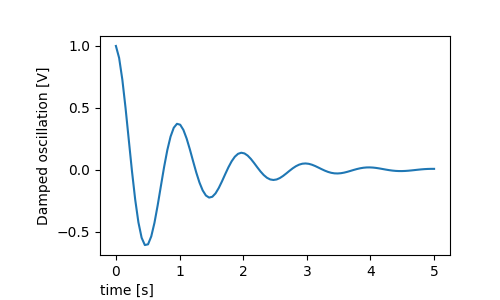All the labelling in this tutorial can be changed by manipulating the matplotlib.font_manager.FontProperties method, or by named kwargs to set_xlabel

from matplotlib.font_manager import FontProperties

font = FontProperties()
font.set_family('serif')
font.set_name('Times New Roman')
font.set_style('italic')

fig, ax = plt.subplots(figsize=(5, 3))
fig.subplots_adjust(bottom=0.15, left=0.2)
ax.plot(x1, y1)
ax.set_xlabel('time [s]', fontsize='large', fontweight='bold')
ax.set_ylabel('Damped oscillation [V]', fontproperties=font)

plt.show()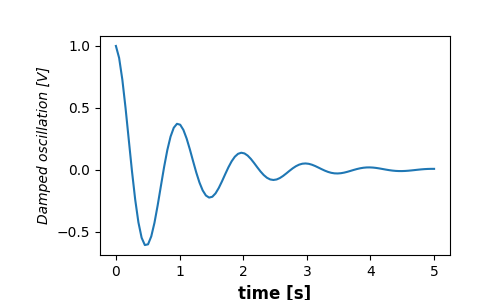Finally, we can use native TeX rendering in all text objects and have multiple lines:

fig, ax = plt.subplots(figsize=(5, 3))
fig.subplots_adjust(bottom=0.2, left=0.2)
ax.plot(x1, np.cumsum(y1**2))
ax.set_xlabel('time [s] \n This was a long experiment')
ax.set_ylabel(r'$\int\ Y^2\ dt\ \ [V^2 s]$')
plt.show()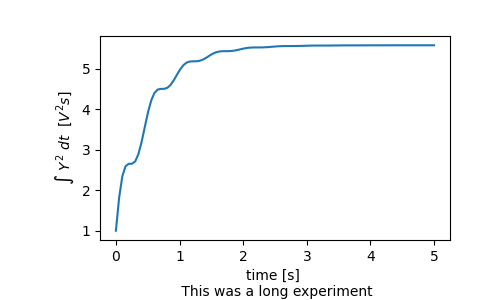## Titles¶

Subplot titles are set in much the same way as labels, but there is the loc keyword arguments that can change the position and justification from the default value of loc=center.

fig, axs = plt.subplots(3, 1, figsize=(5, 6), tight_layout=True)
locs = ['center', 'left', 'right']
for ax, loc in zip(axs, locs):
ax.plot(x1, y1)
ax.set_title('Title with loc at '+loc, loc=loc)
plt.show()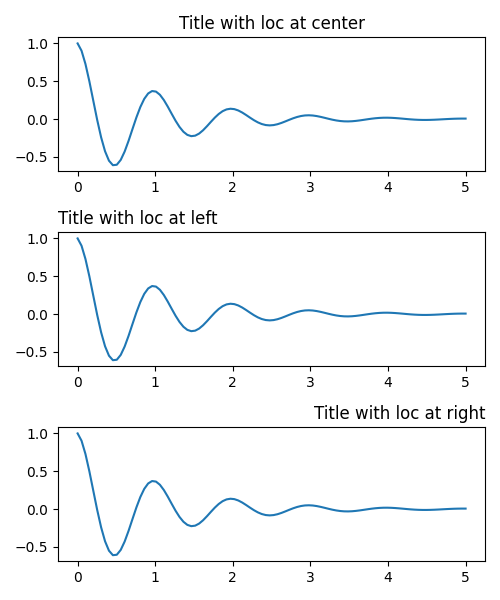Vertical spacing for titles is controlled via rcParams["axes.titlepad"] (default: 6.0), which defaults to 5 points. Setting to a different value moves the title.

fig, ax = plt.subplots(figsize=(5, 3))
fig.subplots_adjust(top=0.8)
ax.plot(x1, y1)
ax.set_title('Vertically offset title', pad=30)
plt.show()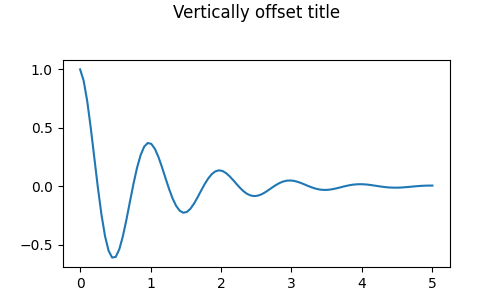## Ticks and ticklabels¶

Placing ticks and ticklabels is a very tricky aspect of making a figure. Matplotlib does its best to accomplish the task automatically, but it also offers a very flexible framework for determining the choices for tick locations, and how they are labelled.

### Terminology¶

Axes have an matplotlib.axis.Axis object for the ax.xaxis and ax.yaxis that contain the information about how the labels in the axis are laid out.

The axis API is explained in detail in the documentation to axis.

An Axis object has major and minor ticks. The Axis has Axis.set_major_locator and Axis.set_minor_locator methods that use the data being plotted to determine the location of major and minor ticks. There are also Axis.set_major_formatter and Axis.set_minor_formatter methods that format the tick labels.

### Simple ticks¶

It often is convenient to simply define the tick values, and sometimes the tick labels, overriding the default locators and formatters. This is discouraged because it breaks interactive navigation of the plot. It also can reset the axis limits: note that the second plot has the ticks we asked for, including ones that are well outside the automatic view limits.

fig, axs = plt.subplots(2, 1, figsize=(5, 3), tight_layout=True)
axs.plot(x1, y1)
axs.plot(x1, y1)
axs.xaxis.set_ticks(np.arange(0., 8.1, 2.))
plt.show()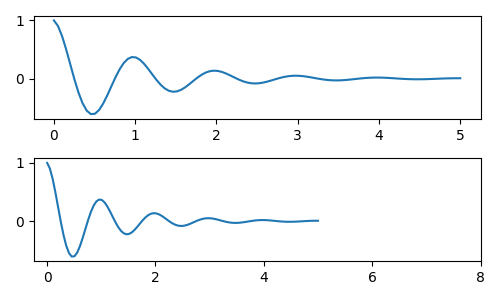We can of course fix this after the fact, but it does highlight a weakness of hard-coding the ticks. This example also changes the format of the ticks:

fig, axs = plt.subplots(2, 1, figsize=(5, 3), tight_layout=True)
axs.plot(x1, y1)
axs.plot(x1, y1)
ticks = np.arange(0., 8.1, 2.)
# list comprehension to get all tick labels...
tickla = [f'{tick:1.2f}' for tick in ticks]
axs.xaxis.set_ticks(ticks)
axs.xaxis.set_ticklabels(tickla)
axs.set_xlim(axs.get_xlim())
plt.show()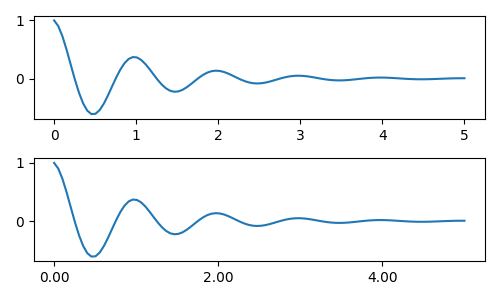### Tick Locators and Formatters¶

Instead of making a list of all the tickalbels, we could have used matplotlib.ticker.StrMethodFormatter (new-style str.format() format string) or matplotlib.ticker.FormatStrFormatter (old-style '%' format string) and passed it to the ax.xaxis. A matplotlib.ticker.StrMethodFormatter can also be created by passing a str without having to explicitly create the formatter.

fig, axs = plt.subplots(2, 1, figsize=(5, 3), tight_layout=True)
axs.plot(x1, y1)
axs.plot(x1, y1)
ticks = np.arange(0., 8.1, 2.)
axs.xaxis.set_ticks(ticks)
axs.xaxis.set_major_formatter('{x:1.1f}')
axs.set_xlim(axs.get_xlim())
plt.show()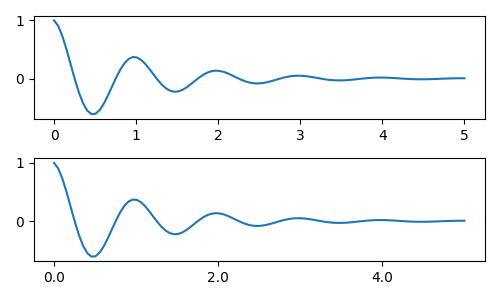And of course we could have used a non-default locator to set the tick locations. Note we still pass in the tick values, but the x-limit fix used above is not needed.

fig, axs = plt.subplots(2, 1, figsize=(5, 3), tight_layout=True)
axs.plot(x1, y1)
axs.plot(x1, y1)
locator = matplotlib.ticker.FixedLocator(ticks)
axs.xaxis.set_major_locator(locator)
axs.xaxis.set_major_formatter('±{x}°')
plt.show()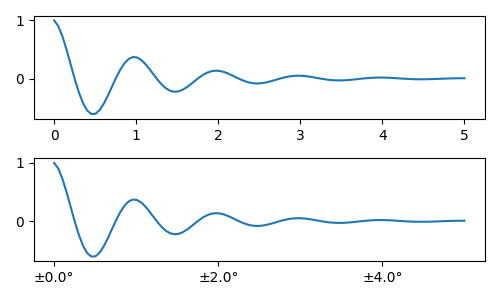The default formatter is the matplotlib.ticker.MaxNLocator called as ticker.MaxNLocator(self, nbins='auto', steps=[1, 2, 2.5, 5, 10]) The steps keyword contains a list of multiples that can be used for tick values. i.e. in this case, 2, 4, 6 would be acceptable ticks, as would 20, 40, 60 or 0.2, 0.4, 0.6. However, 3, 6, 9 would not be acceptable because 3 doesn't appear in the list of steps.

nbins=auto uses an algorithm to determine how many ticks will be acceptable based on how long the axis is. The fontsize of the ticklabel is taken into account, but the length of the tick string is not (because its not yet known.) In the bottom row, the ticklabels are quite large, so we set nbins=4 to make the labels fit in the right-hand plot.

fig, axs = plt.subplots(2, 2, figsize=(8, 5), tight_layout=True)
for n, ax in enumerate(axs.flat):
ax.plot(x1*10., y1)

formatter = matplotlib.ticker.FormatStrFormatter('%1.1f')
locator = matplotlib.ticker.MaxNLocator(nbins='auto', steps=[1, 4, 10])
axs[0, 1].xaxis.set_major_locator(locator)
axs[0, 1].xaxis.set_major_formatter(formatter)

formatter = matplotlib.ticker.FormatStrFormatter('%1.5f')
locator = matplotlib.ticker.AutoLocator()
axs[1, 0].xaxis.set_major_formatter(formatter)
axs[1, 0].xaxis.set_major_locator(locator)

formatter = matplotlib.ticker.FormatStrFormatter('%1.5f')
locator = matplotlib.ticker.MaxNLocator(nbins=4)
axs[1, 1].xaxis.set_major_formatter(formatter)
axs[1, 1].xaxis.set_major_locator(locator)

plt.show()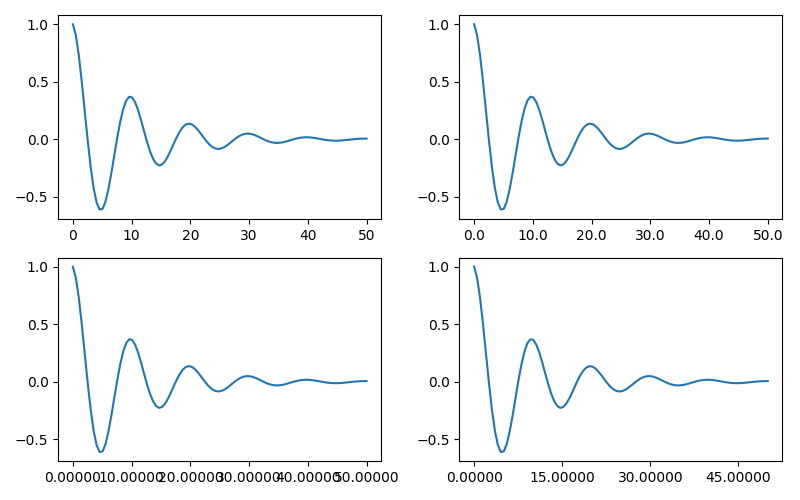Finally, we can specify functions for the formatter using matplotlib.ticker.FuncFormatter. Further, like matplotlib.ticker.StrMethodFormatter, passing a function will automatically create a matplotlib.ticker.FuncFormatter.

def formatoddticks(x, pos):
"""Format odd tick positions."""
if x % 2:
return f'{x:1.2f}'
else:
return ''

fig, ax = plt.subplots(figsize=(5, 3), tight_layout=True)
ax.plot(x1, y1)
locator = matplotlib.ticker.MaxNLocator(nbins=6)
ax.xaxis.set_major_formatter(formatoddticks)
ax.xaxis.set_major_locator(locator)

plt.show()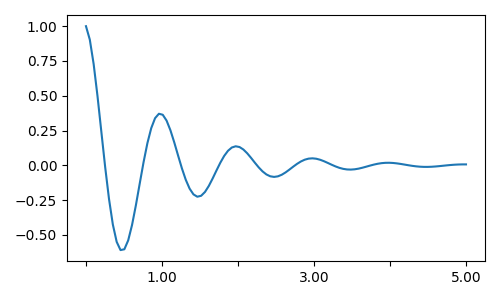### Dateticks¶

Matplotlib can accept datetime.datetime and numpy.datetime64 objects as plotting arguments. Dates and times require special formatting, which can often benefit from manual intervention. In order to help, dates have special Locators and Formatters, defined in the matplotlib.dates module.

A simple example is as follows. Note how we have to rotate the tick labels so that they don't over-run each other.

import datetime

fig, ax = plt.subplots(figsize=(5, 3), tight_layout=True)
base = datetime.datetime(2017, 1, 1, 0, 0, 1)
time = [base + datetime.timedelta(days=x) for x in range(len(x1))]

ax.plot(time, y1)
ax.tick_params(axis='x', rotation=70)
plt.show()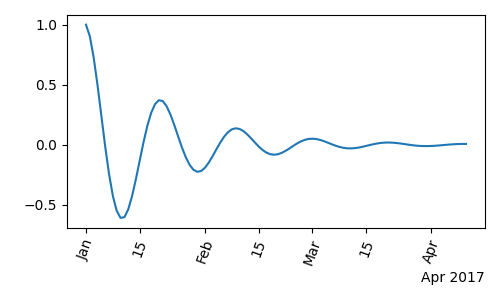We can pass a format to matplotlib.dates.DateFormatter. Also note that the 29th and the next month are very close together. We can fix this by using the dates.DayLocator class, which allows us to specify a list of days of the month to use. Similar formatters are listed in the matplotlib.dates module.

import matplotlib.dates as mdates

locator = mdates.DayLocator(bymonthday=[1, 15])
formatter = mdates.DateFormatter('%b %d')

fig, ax = plt.subplots(figsize=(5, 3), tight_layout=True)
ax.xaxis.set_major_locator(locator)
ax.xaxis.set_major_formatter(formatter)
ax.plot(time, y1)
ax.tick_params(axis='x', rotation=70)
plt.show()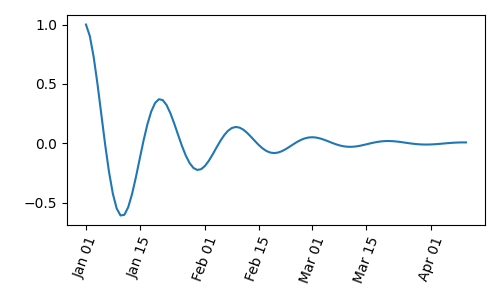## Legends and Annotations¶

Total running time of the script: ( 0 minutes 4.816 seconds)

Keywords: matplotlib code example, codex, python plot, pyplot Gallery generated by Sphinx-Gallery